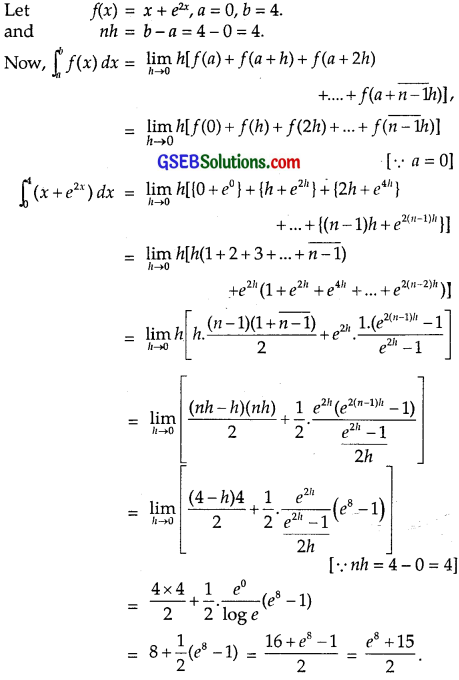# GSEB Solutions Class 12 Maths Chapter 7 Integrals Ex 7.8

Gujarat Board GSEB Textbook Solutions Class 12 Maths Chapter 7 Integrals Ex 7.8 Textbook Questions and Answers.

## Gujarat Board Textbook Solutions Class 11 Maths Chapter 7 Integrals Ex 7.8

Evaluate the following definite integrals as limits of the sum:
Question 1.
$$\int_{a}^{b}$$ x dx
Solution: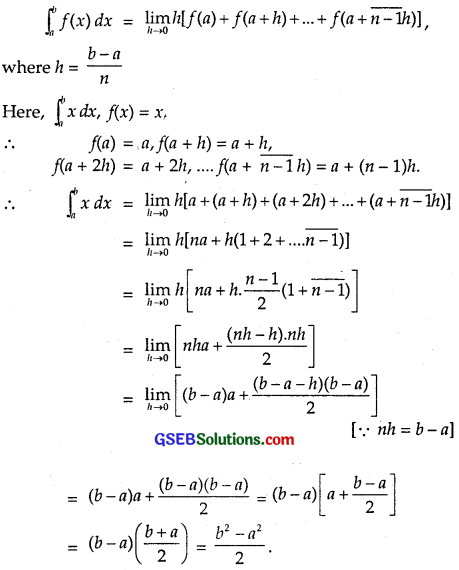Question 2.
$$\int_{5}^{0}$$ (x + 1) dx
Solution:
Compare $$\int_{5}^{0}$$ (x + 1) dx with $$\int_{b}^{a}$$ f(x) dx, we have:
f(x) = x + 1, ‘a’ = 0, ‘b’ = 5 and nh = b – a = 5 – 0 = 5.
Now, f(a) = f(0) = 0 + 1 = 1
f(a + h) = f(h) = h + 1 = 1 + h
f(a + 2h) = f(2h) = 2h + 1 = 1 + 2h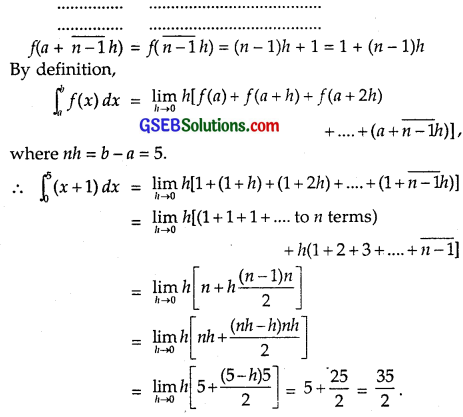Question 3.
$$\int_{-3}^{2}$$ x2 dx
Solution: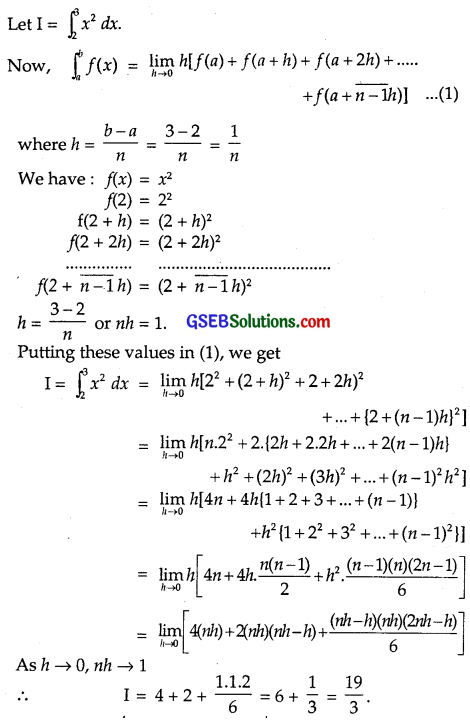Question 4.
$$\int_{-4}^{1}$$ (x2 – x) dx
Solution: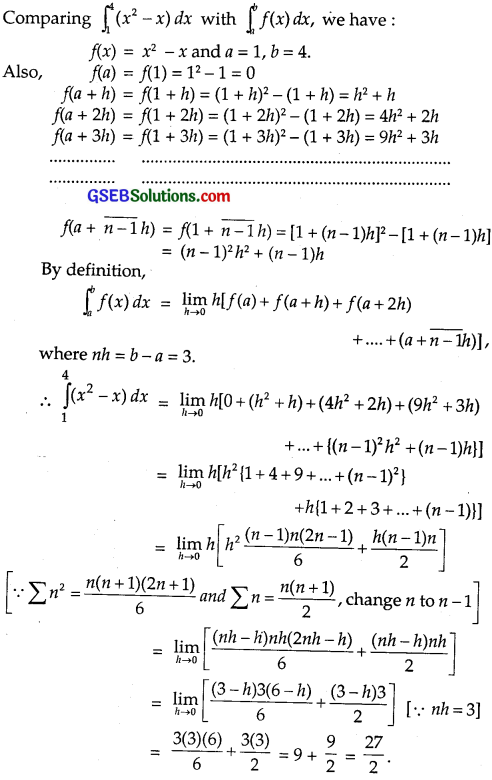Question 5.
$$\int_{1}^{-1}$$ ex dx
Solution: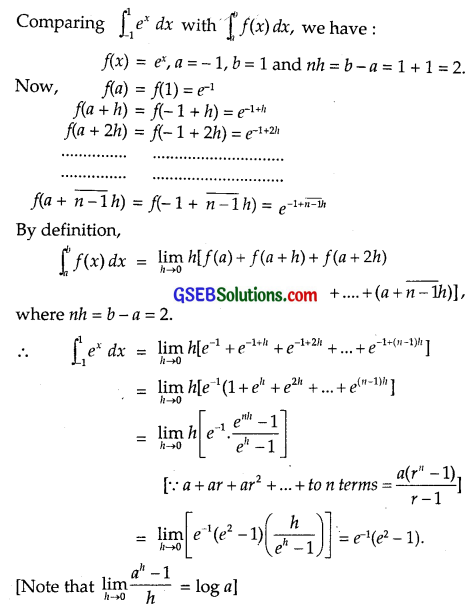Question 6.
$$\int_{4}^{0}$$ (x + e2x) dx
Solution: